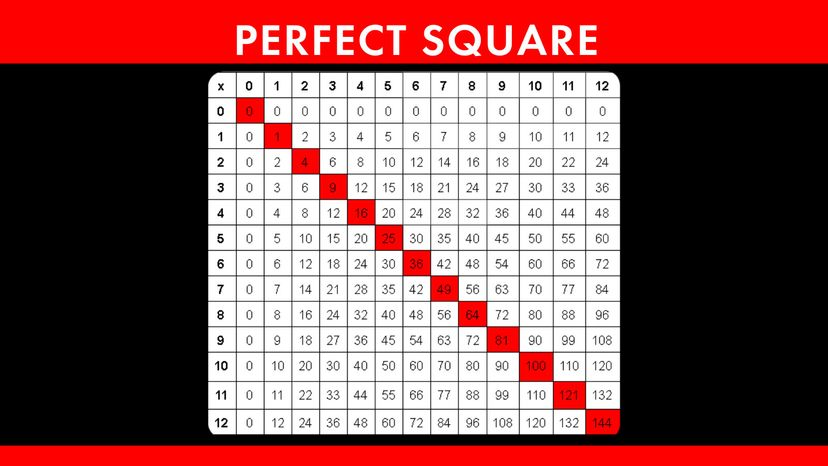# What Is a Perfect Square?

By: Jesslyn Shields  |A perfect square is a number you get by multiplying another number by itself. HowStuffWorks

You know what a square is: It's a shape with four equal sides. Seems hard to improve upon, right? What, then, is a perfect square? In order to explain that, we'll have to get a little math-y.

"Square" is one of those words that can refer to a shape, but it can also mean multiplying a number by itself. It's a little bit like an actual square because if you drew a square on graph paper, each side would take up the same number of units: A square that takes up five horizontal units would also take up five vertical units. If you counted up all the units of graph paper taken up by that particular square, you would find there were 25. Because 5 x 5 = 25.

A "perfect square" refers to a type of number. Much like a prime number is a number that can't be made by simply multiplying two other whole numbers together (a prime number is a positive number greater than 1 that can only be divided by 1 or by itself), a perfect square is a number you get by multiplying another number by itself. For instance, 16 is a perfect square because you get it by multiplying 4 by 4; 144 is a perfect square because it can be achieved by multiplying 12 by itself.

So, how do you know if a number is a perfect square? You can do that by finding its square root, which is the opposite of squaring a number. If the square root is a whole number, then it's a perfect square.